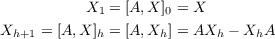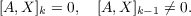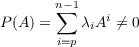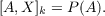#### Vol. 32, No. 1, 1970

 Download this articleFor screen For printingRecent Issues Vol. 325: 1 Vol. 324: 1  2 Vol. 323: 1  2 Vol. 322: 1  2 Vol. 321: 1  2 Vol. 320: 1  2 Vol. 319: 1  2 Vol. 318: 1  2Online Archive Volume: Issue:The Journal Subscriptions Editorial Board Officers Contacts Submission Guidelines Submission Form Policies for Authors ISSN: 1945-5844 (e-only) ISSN: 0030-8730 (print) Special Issues Author Index To Appear Other MSP Journals
Matric polynomials which are higher commutators

### Edmond Dale Dixon

Vol. 32 (1970), No. 1, 55–63
##### Abstract

Let A be an n × n matrix defined over a field F of characteristic greater than n. For each n × n matrix X we define(1)

for each positive integer h. Then X is defined to be k-commutative with A if and only if(2)

Let P(x) be a polynomial such that P(A)0. Specifically, assume that(3)

where p is a positive integer, each λi is a scalar from F, and λp0. In this paper we study, for each positive integer k, the matrices X such that(4)

We specify a polynomial P(A) in the form (3) and show how the maximal value of k for which (4) has a solution depends on the polynomial P(A). In Theorem 3 it is assumed that A is nonderogatory. Since the only matrices which commute with A in this case are polynomials in A, we are, in effect, establishing a more precise bound for k in (2) by predetermining Xk.

In the derogatory case, a matrix which is not a polynomial in A may commute with A. However, Theorem 4 shows that if we choose a polynomial P(A) as Xk, then the maximal value of k depends on the polynomial P.

Primary: 15.35
##### Milestones